Next: Summary and Properties Up: No Title Previous: Imaging Geometry

# 3x4 Projection Matrix

There are three coordinate systems involved --- camera, image and world.

1. Camera: perspective projection.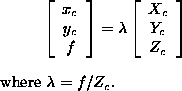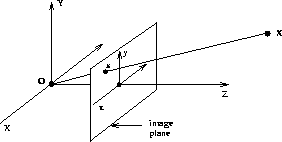This can be written as a linear mapping between homogeneous coordinates (the equation is only up to a scale factor):where a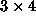projection matrix represents a map from 3D to 2D.

2. Image: (intrinsic/internal camera parameters)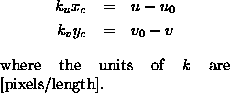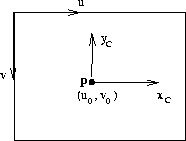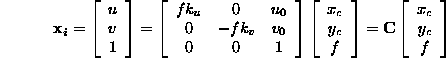is a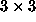upper triangular matrix, called the camera calibration matrix: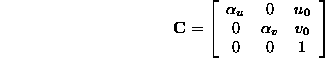where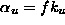,.

•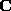provides the transformation between an image point and a ray in Euclidean 3-space.
• There are four parameters:
1. The scaling in the image x and y directions,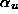and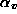.
2. The principal point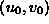, which is the point where the optic axis intersects the image plane.
The aspect ratio is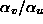.
• Onceis known the camera is termed calibrated.
• A calibrated camera is a direction sensor, able to measure the direction of rays --- like a 2D protractor.

3. World: (extrinsic/external camera parameters)
The Euclidean transformation between the camera and world coordinates is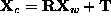: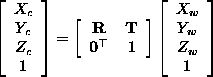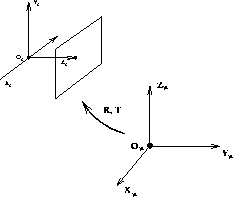Finally, concatenating the three matrices,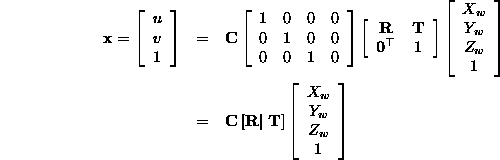which defines theprojection matrix from Euclidean 3-space to an image:

###Bob Fisher
Wed Apr 16 00:58:54 BST 1997